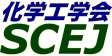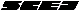## \$B9V1i%W%m%0%i%`!J2q>l!&F|DxJL!K(B

### H\$B2q>l(B \$BBh(B1\$BF|(B

\$B:G=*99?7F|;~!'(B2012-08-20 15:02:17
\$B9V1i(B
\$B;~9o(B
\$B9V1i(B
\$BHV9f(B
\$B9V1iBjL\!?H/I=\$B%-!<%o!<%I(B\$BJ,N`(B
\$BHV9f(B
\$B\$BHV9f(B
\$B%7%s%]%8%&%`(B \$B!c;q8;!&%(%M%k%.!
(9:00\$B!A(B10:00)\$B!!(B(\$B:BD9(B \$BHx>e(B \$B70(B)
9:00\$B!A(B 9:20H101\$B!HD65[Ce
(\$B6eBg?eAG%;(B) \$B!{(B(\$B@5(B)\$B>.AR(B \$BE4J?(B \$B!&(B (\$B6eBg1!9)(B) \$B7n@n(B \$B5W5A(B \$B!&(B \$BEDEg(B \$B@54n(B
High-Temperature Surface
Density Functional Theory
S-5149
9:20\$B!A(B 9:40H102Pt-Rh\$B?(G^\$G\$NGS%,%9>t2=H?1~\$NH?1~9)3XE*%b%G%j%s%0(B
(\$B5~Bg9)(B) \$B!{(B(\$B3X(B)\$B2,(B \$BMN51(B \$B!&(B (\$B3X(B)\$B5HLnC+(B \$BBs:H(B \$B!&(B (\$B@5(B)\$B0f>e(B \$B85(B \$B!&(B (ICT) \$B5H@n(B \$BN5Lo(B \$B!&(B (\$B5~Bg9)(B) (\$B@5(B)\$B2O@%(B \$B85L@(B
Automotive exhaust gas
Pt-Rh catalyst
Reaction modeling
S-5268
9:40\$B!A(B 10:00H103\$BA+0\6bB0;@2=J*\$rMQ\$\$\$?(BVOC\$B;@2=>t2=MQ?(G^\$K4X\$9\$k8&5f(B
(\$BElG@9)Bg(B) \$B!{(B(\$B3X(B)\$BlK(B \$B\$m(B \$B!&(B (\$B@5(B)\$B3T(B \$B%f(B \$B!&(B (\$B@5(B)\$B:y0f(B \$B@?(B \$B!&(B (\$B@5(B)\$B55;3(B \$B=(M:(B
Catalytic Oxidation
Volatile Organic Compounds
Synergistic Effect
S-574
(10:00\$B!A(B11:00)\$B!!(B(\$B:BD9(B \$B>.AR(B \$BE4J?(B)
10:00\$B!A(B 10:20H104\$B4^%U%CAGM-5!2=9gJ*\$NJ,2r8GDj2=MQ?75,:`NA\$NAO@=(B
(\$B1'ET5\Bg1!9)(B) \$B!{(B(\$B3X(B)\$B>BHx(B \$BBn;V(B \$B!&(B (\$B@5(B)\$B8E_7(B \$B5#(B \$B!&(B \$B>>K\(B \$BB@51(B \$B!&(B (\$B@5(B)\$B:4F#(B \$B@5=((B \$B!&(B (\$B@5(B)\$BNkLZ(B \$B>:(B
CaO
VOC
SiO\$2
S-5276
10:20\$B!A(B 10:40H105\$BF<%\$%*%s\$K\$h\$k%U%'%s%H%s;@2=\$NB%?J5!9=\$N8!F\$(B
(\$B5~Bg9)(B) \$B!{(B(\$B3X(B)\$BA0@n(B \$B=_(B \$B!&(B (\$B@5(B)\$BA0(B \$B0lW"(B \$B!&(B (\$B5~Bg4D2J%;(B) (\$B@5(B)\$BCf@n(B \$B9@9T(B
fenton reaction
copper ion
mineralization
S-5296
10:40\$B!A(B 11:00H106\$B%;%k%m!<%9\$NB?CJ=hM}\$K\$h\$k%l%V%j%s;@\$N@=B\$(B
(\$B5~Bg9)(B) \$B!{(B(\$B3X(B)\$BB \$B!&(B (\$B@5(B)\$BD9C+@n(B \$B8y(B \$B!&(B (\$B@5(B)\$BA0(B \$B0lW"(B
cellulose
levulinic acid
pre-treatment
S-5100
\$B%7%s%]%8%&%`(B \$B!cH?1~9)3XIt2q%]%9%?!<%;%C%7%g%s!d(B
(11:00\$B!A(B12:00)\$B!!(B(\$B:BD9(B \$B5WJ](B \$B@5
11:00\$B!A(B 11:20XC1P01\$B%R!<%H%3%s%P%\$%s%I7??(G^H?1~%7%9%F%`\$K\$h\$k%a%?%s2~
(\$B@EBg1!(B) \$B!{(B(\$B3X(B)\$B<,;3(B \$BM:Bg(B \$B!&(B \$BH*(B \$BB@5.(B \$B!&(B (\$B@EBg9)(B) (\$B@5(B)\$BEOIt(B \$B0=(B \$B!&(B (\$B@5(B)\$BJ!86(B \$BD9
Process intensification
Methane steam reforming
Structured catalyst
S-9563
11:00\$B!A(B 11:20XC1P02Pt/TiO2\$B?(G^\$K\$h\$k%K%H%m%9%A%l%s?eAG2=(B -\$B7k>=;R7B\$H(BN\$B%I!<%W\$N1F6A(B-
(\$BKLBg1!9)(B) \$B!{(B(\$B3X(B)\$BK'ED(B \$B2E;V(B \$B!&(B \$B8^==Mr(B \$B>0Li(B \$B!&(B (\$B@5(B)\$BF#ED(B \$B?J0lO:(B \$B!&(B (\$B@5(B)\$B9S0f(B \$B@5I'(B
supported metal catalyst
crystallite size
nitrostyrene
S-9345
11:00\$B!A(B 11:20XC1P03\$B%Q%i%8%&%`?(G^\$K\$h\$k%W%m%T%*%s%"%k%G%R%I\$N;@2=E*%(%9%F%k2=H?1~\$KBP\$9\$kC4BN8z2L(B
(\$BFAEgBg1!@hC<650iIt(B) \$B!{(B(\$B3X(B)\$B:dEl(B \$B9*Ln(B \$B!&(B (\$BFAEgBg9)(B) \$BEOJU(B \$B3(H~;R(B \$B!&(B (\$BFAEgBg1!(BSTS\$B8&5fIt(B) (\$B@5(B)\$BCf@n(B \$B7I;0(B \$B!&(B (\$B@5(B)\$B2CF#(B \$B2mM5(B \$B!&(B (\$B@5(B)\$B30NX(B \$B7r0lO:(B \$B!&(B (\$B@5(B)\$B?y;3(B \$BLP(B \$B!&(B (\$B;0I)%l%\$%h%s(B) \$B2CF#(B \$BM5 \$B!&(B \$B=)86(B \$B=(<#(B \$B!&(B \$BFs5\(B \$B9R(B
Pd-supported catalyst
Oxidative esterification
Methyl propionate
S-9328
11:00\$B!A(B 11:20XC1P04\$B@=K!\$N0[\$J\$k(BPSS\$B;Y;}BN\$K@=Kl\$5\$l\$?(BPd\$BKl\$rMQ\$\$\$?%(%?%N!<%k\$N%9%A!<%`%j%U%)!<%_%s%0(B
(\$BFAEgBg1!@hC<650iIt(B) \$B!{(B(\$B3X(B)\$B@P0f(B \$B0!M}:;(B \$B!&(B (\$B3X(B)\$BLZFb(B \$B9@Fs(B \$B!&(B \$B3@Fb(B \$BC#Li(B \$B!&(B \$BKL86(B \$BM'N<(B \$B!&(B (\$BFAEgBg1!(BSTS\$B8&5fIt(B) (\$B@5(B)\$B2CF#(B \$B2mM5(B \$B!&(B (\$B@5(B)\$BKY2O(B \$B=S1Q(B \$B!&(B (\$B@5(B)\$BCf@n(B \$B7I;0(B \$B!&(B (\$B@5(B)\$B?y;3(B \$BLP(B
Hydrogen permeability
Ethanol steam reforming
S-9331
11:00\$B!A(B 11:20XC1P05\$B%;%j%"%J%N%m%C%I\$N;@2=?(G^3h@-\$K5Z\$\\$93F
(\$BFAEgBg1!@hC<650iIt(B) \$B!{(B(\$B3X(B)\$BBgEg(B \$BBnLi(B \$B!&(B (\$B3X(B)\$BJR;3(B \$B7C(B \$B!&(B (\$BFAEgBg1!(BSTS\$B8&(B) (\$B@5(B)\$BCf@n(B \$B7I;0(B \$B!&(B (\$B@5(B)\$B2CF#(B \$B2mM5(B \$B!&(B (\$B@5(B)\$B30NX(B \$B7r0lO:(B \$B!&(B (\$B@5(B)\$B?y;3(B \$BLP(B
cerium oxide
supported catalysts
carbon combustion
S-9462
11:00\$B!A(B 11:20XC1P06\$BL)EYHF4X?tK!\$rMQ\$\$\$?(BTiO2\$B8w?(G^\$K\$h\$k%"%s%b%K%"8w?(G^J,2rH?1~\$N5!9=2rL@(B
(\$B?@8MBg1!9)(B) \$B!{(B(\$B3X(B)\$B@>Ln(B \$B9,9-(B \$B!&(B (\$B3X(B)\$BKL@n(B \$B7r2p(B \$B!&(B (\$B3X(B)\$B;38}(B \$B\$a\$0\$_(B \$B!&(B (\$B5~9)A!1!9)(B) \$B>.NS(B \$B5WK'(B \$B!&(B (\$B?@8MBg1!9)(B) (\$B@5(B)\$B;T66(B \$BM40l(B \$B!&(B (\$B@5(B)\$B@>;3(B \$B3P(B
density functional theory
photodecomposition of NH3
photocatalyst
S-9613
11:00\$B!A(B 11:20XC1P07\$B8w?(G^J,2rH?1~\$K\$*\$1\$k?(G^G;EY\$NH?1~B.EY\$KM?\$(\$k1F6A(B
(\$B?@8MBg1!9)(B) \$B!{(B(\$B3X(B)\$BlK(B \$BG+(B \$B!&(B (\$B@5(B)\$B7'C+(B \$B595W(B \$B!&(B (\$B@5(B)\$BKY9>(B \$B9';K(B \$B!&(B (\$B@5(B)\$BBgB<(B \$BD>?M(B
Photocatalyst
Decomposition rate
Catalyst concentration
S-9628
11:00\$B!A(B 11:20XC1P08\$B1UBN3&LL\$rMxMQ\$7\$?%W%i%:%^2=3XH?1~\$K\$h\$k%P%\$%*%^%9=hM}(B
(\$B6bBtBgM}9)8&5f0h(B) \$B!{(B(\$B3X(B)\$B;3@%(B \$BN<(B \$B!&(B (\$B3X(B)\$B9u_7(B \$BCNN\$(B \$B!&(B \$B2C2l(B \$B=S \$B!&(B \$B@PEg(B \$BC#IW(B \$B!&(B (\$B@5(B)\$B9b66(B \$B7{;J(B
plasma
biomass
S-9578
11:00\$B!A(B 11:20XC1P09\$B%\$%*%s1UBN\$rMQ\$\$\$?%P%\$%*%^%9%j%U%!%\$%J%j!<(B
(\$B6bBtBg1!M}9)8&5f0h(B) \$B!{(B(\$B3X(B)\$B@D?9(B \$BM-9a(B \$B!&(B (\$B6bBtBgM}9)3X0h(B) \$B0f>e(B \$B7r(B \$B!&(B \$B@>_7(B \$BM*4u(B \$B!&(B (\$B6bBtBg4DF|K\3\$(B) (\$B@5(B)\$B?N5\(B \$B0l>O(B \$B!&(B (\$B6bBtBgM}9)3X8&5f0h(B) (\$B@5(B)\$B9b66(B \$B7{;J(B
biorefinery
lignocellulose
ionic liquid
S-9580
11:00\$B!A(B 11:20XC1P10\$B%"%s%b%K%"J,2r\$K\$*\$1\$kH?1~B.EY%b%G%k\$H?eAGJ,N%8z2L(B
(\$B1'ET5\Bg1!9)(B) \$B!{(B(\$B3X(B)\$B?\2l(B \$B1QB@(B \$B!&(B (\$B@5(B)\$B:4F#(B \$B9d;K(B \$B!&(B (\$B@5(B)\$B0KF#(B \$BD>
ammonia
kinetics
hydrogen
S-9281
11:20\$B!A(B 11:40XC1P11\$BH?1~J,N%\$rMQ\$\$\$?%U%'%N!<%k\$N0lCJ9g@.%7%9%F%`\$K\$*\$1\$kAK32M^@)>r7o\$N8!F\$(B
(\$BL>Bg1!9)(B) \$B!{(B(\$B3X(B)\$B@nB<(B \$BBYGn(B \$B!&(B (\$B@5(B)\$BED@n(B \$BCRI'(B \$B!&(B (\$B@5(B)\$B;3ED(B \$BGn;K(B
phenol
partial oxidation
reaction-separation
S-9614
11:20\$B!A(B 11:40XC1P12\$B%V%?%N!<%k\$rG3NA8;\$H\$7\$?(BCRGT\$B\$N%7%9%F%`2r@O\$H4XO"MWAG5;=Q\$N4pAC8&5f(B
(\$BL>Bg1!9)(B) \$B!{(B(\$B3X(B)\$BLnF~(B \$BMNN<(B \$B!&(B (\$BL>Bg9)(B) \$BI)>B(B \$B5.G7(B \$B!&(B (\$BL>Bg1!9)(B) \$B:4F#(B \$B2mI'(B \$B!&(B (\$BL>Bg%(%3%H%T%"(B) (\$B@5(B)\$B>.Eg(B \$B5A90(B
gas turbine
butanol
steam reforming
S-9900
11:20\$B!A(B 11:40XC1P13\$B%^%\$%/%mN.O)\$rMQ\$\$\$?%F%"%K%s\$NO"B3@8;:\$K\$*\$1\$k29EY!&(BpH\$B\$N1F6A(B
(\$B;:Am8&(B) \$B!{(B(\$B@5(B)\$B>>1:(B \$B=S0l(B \$B!&(B \$B30Lg(B \$B7CH~;R(B \$B!&(B \$B@iMU(B \$B??F`H~(B \$B!&(B \$B3QED(B \$BC#O/(B
microreactor
mesoporous silica
theanine
S-971
11:20\$B!A(B 11:40XC1P14\$BN.BNNO3XE*%-%c%S%F!<%7%g%s\$rMxMQ\$7\$?AuCV\$N3+H/(B
(\$BL>Bg1!9)(B) \$B!{(B(\$B3X(B)\$BCCLj(B \$BEl0!(B \$B!&(B (\$B@5(B)\$B0BED(B \$B7<;J(B
cavitation
microbubble
hydrodynamics
S-9203
11:20\$B!A(B 11:40XC1P15A study of sonochemical reactors at low frequency
(Nagoya U., Grad. Sch. of Eng.) \$B!{(B(\$B3X(B)Tran Khuyen Viet Bao \$B!&(B (Honda Electronics Co.) (\$B@5(B)Asakura Yoshiyuki \$B!&(B (Nagoya U., Grad. Sch. of Eng.) (\$B@5(B)Koda Shinobu
Sonochemical reactor
chemical effects
mechanical effects
S-9683
11:20\$B!A(B 11:40XC1P16\$BD62;GHCf6u%[!<%s\$H%^%\$%/%m%P%V%k\$rMQ\$\$\$?%\$%s%8%4%+%k%_%sJ,2r%W%m%;%9(B
(\$B;37ABg9)(B) \$B!{(B(\$B3X(B)\$BAj_7(B \$BM%B@(B \$B!&(B (\$B;37ABg1!M}9)(B) (\$B@5(B)\$BKkED(B \$B
Sonochemistry
Ultrasonic horn
Microbubble
S-956
11:20\$B!A(B 11:40XC1P17\$BD62;GH%*%>%s%^%\$%/%m%P%V%k\$rMQ\$\$\$?%j%0%K%s\$NJ,2r(B
(\$B;37ABg9)(B) \$B!{(B(\$B3X(B)\$B9b66(B \$BM[;R(B \$B!&(B (\$B;37ABg1!M}9)(B) (\$B@5(B)\$BKkED(B \$B \$B!&(B \$B;VB<(B \$BNI0lO:(B \$B!&(B \$B \$B!&(B \$B5\ED(B \$B7u(B \$B!&(B \$B9aED(B \$BCRB'(B \$B!&(B \$B@>2,(B \$B>
lignin
microbubbles
ultrasonic hone
S-9857
11:20\$B!A(B 11:40XC1P18\$B=V4V9E2=@-Z(B
(\$B;37ABg9)(B) \$B!{(B(\$B3X(B)\$B:d8}(B \$B2m4u(B \$B!&(B (\$B;37ABgM}9)(B) (\$B@5(B)\$BKkED(B \$B
microbubble
hollow microcapsule
cyanoacrylate resin
S-957
11:20\$B!A(B 11:40XC1P19\$B%^%\$%/%m%P%V%k\$N052u\$rMxMQ\$7\$??'AG4^M-?e\$NJ,2r=hM}\$K4X\$9\$k8&5f(B
(\$B4X@>Bg(B) \$B!{(B(\$B3X(B)\$B?@ED(B \$BHK4u(B \$B!&(B \$B2CLg(B \$B5A?M(B \$B!&(B (\$B@5(B)\$B2,ED(B \$BK'
microbubble
methylene blue
S-9730
11:20\$B!A(B 11:40XC1P20\$B93;@2=?'AG\$K\$h\$k6bB0:xBN\$r7A@.\$9\$k2=3XNLO@Hf\$N8!F\$(B
(\$B@iMU9)Bg9)(B) \$B!{(B(\$B3X(B)\$B>>K\(B \$B??@,(B \$B!&(B \$B2<6?(B \$B9,;R(B \$B!&(B \$BJ!ED(B \$BH~F`(B \$B!&(B \$B;3ED(B \$B%5%A;R(B \$B!&(B (\$B@5(B)\$BHx>e(B \$B70(B
Anthocyanin
Metal complex
Stoichiometric proportion
S-9612
11:40\$B!A(B 12:00XC1P21\$B%3!<%k%I%&%)!<%k7?G.(BCVD\$BAuCV\$rMQ\$\$\$?;@2=0!1t\$N@.Kl\$HH?1~2r@O(B
(\$BEl3\$Bg(B) \$B!{(B(\$B3X(B)\$BBg5WJ](B \$BC#@8(B \$B!&(B (\$B3X(B)\$BEl(B \$BM%4u(B \$B!&(B (\$B3X(B)\$B5H_7(B \$BM5Li(B \$B!&(B \$B^I9a(B \$BN4(B \$B!&(B (\$B@5(B)\$B=);3(B \$BBY?-(B
CVD
Zinc oxide
CW
S-9739
11:40\$B!A(B 12:00XC1P22\$B%"%;%A%k%"%;%H%J!<%H0!1t\$r86NA\$H\$7\$?;@2=0!1tGvKl\$N8:05G.(BCVD
(\$BEl3\$Bg(B) \$B!{(B(\$B3X(B)\$BLn2<(B \$B=_(B \$B!&(B (\$B3X(B)\$B@690(B \$B7k0a(B \$B!&(B \$B^I9a(B \$BN4(B \$B!&(B (\$B@5(B)\$B=);3(B \$BBY?-(B
CVD
Zinc oxide
Zinc acetylacetonate
S-9826
11:40\$B!A(B 12:00XC1P23\$B%j%A%&%`Fs2A(B
(\$BJF;R9b@l(B) \$B!{(B(\$B3X(B)\$B@.ED(B \$B5.8w(B \$B!&(B (\$B3X(B)\$B%h%O%M%9(B \$B%/%j%9%F%#(B \$B!&(B (\$BJF;R9b@l@l962J(B) \$Bzp66(B \$BBg \$B!&(B (\$BJF;R9b@l(B) \$B>>0f(B \$B0jLi(B \$B!&(B \$B@V0f(B \$BBg5\$(B \$B!&(B \$BC]Fb(B \$BM4B@(B \$B!&(B \$BJ?2,(B \$BBsMN(B \$B!&(B (\$B@5(B)\$BC+F#(B \$B>05.(B \$B!&(B (\$BL>Bg1!M}(B) \$B5H@n(B \$B9@;K(B
non solvent reaction
disulfide
lithium rechargable battery
S-9214
11:40\$B!A(B 12:00XC1P24\$B6b%J%NN3;R8GDj2=F3EE@-%+!<%\%s@EEEKB;eIT?%I[\$N3+H/(B
(\$B:eBg1!4p9)(B) \$B!{(B(\$B3X(B)\$B_7ED(B \$B8w0l(B \$B!&(B (\$B@5(B)\$B6-(B \$B?5;J(B \$B!&(B (\$B@5(B)\$BEDC+(B \$B@5?N(B
Electrospinning
Carbon nanofiber
Gold nanoparticle
S-9554
(15:00\$B!A(B15:40)\$B!!(B(\$B:BD9(B \$BHSED(B \$BH%(B)
15:00\$B!A(B 15:20H119\$B3FFH?1~FC@-(B
(\$B6eBg1!9)(B) \$B!{(B(\$B3X(B)\$BEOJU(B \$BM:B@(B \$B!&(B (\$B@5(B)\$B>>:,(B \$B1Q \$B!&(B (\$B@5(B)\$BC]Cf(B \$BAT(B \$B!&(B (\$B@5(B)\$B4_ED(B \$B>;9@(B
Pt catalyst
decane combustion
complex oxide
S-5294
15:20\$B!A(B 15:40H120\$BC:;@%;%j%&%`\$r7PM3\$7\$?%m%C%I>u%;%j%"%J%NN3;R\$ND4@=\$H%a%?%s5Z\$SC:AGG3>FFC@-(B
(\$BFAEgBg1!(BSTS\$B8&(B) \$B!{(B(\$B@5(B)\$BCf@n(B \$B7I;0(B \$B!&(B (\$BFAEgBg1!@hC<650iIt(B) (\$B3X(B)\$BJR;3(B \$B7C(B \$B!&(B (\$B3X(B)\$BBgEg(B \$BBnLi(B \$B!&(B (\$BFAEgBg1!(BSTS\$B8&(B) (\$B@5(B)\$B2CF#(B \$B2mM5(B \$B!&(B (\$B@5(B)\$B30NX(B \$B7r0lO:(B \$B!&(B (\$B@5(B)\$B?y;3(B \$BLP(B
cerium oxide
nanostructured catalysts
carbon combustion
S-5454
(15:40\$B!A(B16:20)\$B!!(B(\$B;J2q(B \$B>e5\(B \$B@.G7(B)
15:40\$B!A(B 16:20H121[\$BE8K>9V1i(B]\$B!!@PL}@:@=\$N?7\$7\$\$J}8~@-!!(B-HS-FCC\$B\$rBj:`\$K(B-
(JX\$BF|9[F|@P%(%M%k%.!<(B) \$B!{H*Cf(B \$B=E?M(B
oil
chemicals
FCC
S-5583
(16:20\$B!A(B17:00)\$B!!(B(\$B;J2q(B \$BHx>e(B \$B70(B)
16:20\$B!A(B 17:00H123[\$BE8K>9V1i(B] \$B2
(\$BF|N)@=:n=j(B) \$B!{F|9b(B \$B@/N4(B
Micro-Bubble
Ozonation
Wastewater Recycle
S-5219
(17:00\$B!A(B17:40)\$B!!(B(\$B;J2q(B \$B?y;3(B \$BLP(B)
17:00\$B!A(B 17:40H125[\$BE8K>9V1i(B]\$BDc4D6-Ii2Y%W%m%T%l%s%*%-%5%\$%I?75,@=B\$K!\$N3+H/(B
(\$B=;M'2=3X(B) \$B!{DT(B \$B=cJ?(B
\$BH?1~%W%m%;%9(B
\$BDc4D6-(B
\$B%W%m%S%l%s%*%-%5%\$%I(B
S-5412

\$B9V1i%W%m%0%i%`(B
\$B2=3X9)3X2q(B \$BBh(B44\$B2s=)5(Bg2q(B(C) 2012 \$B8x1W
Most recent update: 2012-08-20 15:02:17
For more information contact \$B2=3X9)3X2qElKL;YIt(B \$BBh(B44\$B2s=)5(Bg2q(B \$BLd\$\$9g\$;78(B
E-mail: inquiry-44fwww3.scej.org
This page was generated byeasp 2.28; proghtml 2.28c (C)1999-2011 kawase